Problem

# For the ladder network in Fig. 2.104, find I and Req.

For the ladder network in Fig. 2.104, find I and Req.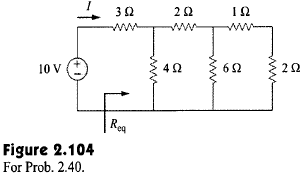#### Step-by-Step Solution

Solution 1

Refer to Figure 2.104 in the text book.

In Figure 2.104, the series combination of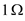and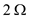resistor is connected in parallel with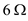resistor.

Calculate the equivalent resistance.Draw the simplified circuit diagram.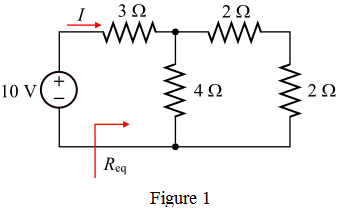In Figure 1, the tworesistors connected in series and this series combination is in parallel with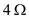resistor.

Calculate the equivalent resistance of series-parallel combination and assign as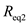.Draw the simplified circuit diagram of Figure 1.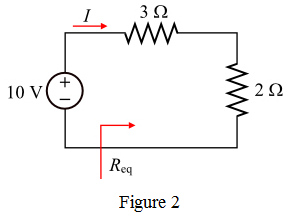In the Figure 2, the resistors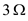andare connected in series.

Calculate the total equivalent resistance,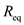.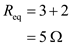Therefore, the equivalent resistance,is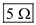.

Calculate the current in the circuit.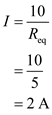Therefore, the current in the circuit,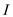is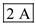.